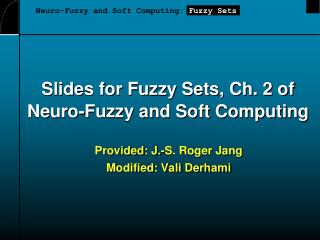DownloadDownload PresentationSlides for Fuzzy Sets, Ch. 2 of Neuro-Fuzzy and Soft Computing

# Slides for Fuzzy Sets, Ch. 2 of Neuro-Fuzzy and Soft Computing

Télécharger la présentation## Slides for Fuzzy Sets, Ch. 2 of Neuro-Fuzzy and Soft Computing

- - - - - - - - - - - - - - - - - - - - - - - - - - - E N D - - - - - - - - - - - - - - - - - - - - - - - - - - -
##### Presentation Transcript

1. Neuro-Fuzzy and Soft Computing: Fuzzy Sets Slides for Fuzzy Sets, Ch. 2 ofNeuro-Fuzzy and Soft Computing • Provided: J.-S. Roger Jang • Modified: Vali Derhami

2. Fuzzy Sets: Outline • Introduction • Basic definitions and terminology • Set-theoretic operations • MF formulation and parameterization • MFs of one and two dimensions • Derivatives of parameterized MFs • More on fuzzy union, intersection, and complement • Fuzzy complement • Fuzzy intersection and union • Parameterized T-norm and T-conorm

3. Crisp set A 1.0 5’10’’ Heights Fuzzy Sets • Sets with fuzzy boundaries A = Set of tall people Fuzzy set A 1.0 .9 Membership function .5 5’10’’ 6’2’’ Heights

4. “tall” in NBA Membership Functions (MFs) • Characteristics of MFs: • Subjective measures • Not probability functions “tall” in Asia MFs .8 “tall” in the US .5 .1 5’10’’ Heights

5. Fuzzy Sets • Formal definition: A fuzzy set A in X is expressed as a set of ordered pairs: Membership function (MF) Universe or universe of discourse Fuzzy set A fuzzy set is totally characterized by a membership function (MF).

6. Fuzzy Sets with Discrete Universes • Fuzzy set C = “desirable city to live in” X = {SF, Boston, LA} (discrete and nonordered) C = {(SF, 0.9), (Boston, 0.8), (LA, 0.6)} • Fuzzy set A = “sensible number of children” X = {0, 1, 2, 3, 4, 5, 6} (discrete universe) A = {(0, .1), (1, .3), (2, .7), (3, 1), (4, .6), (5, .2), (6, .1)}

7. Fuzzy Sets with Cont. Universes • Fuzzy set B = “about 50 years old” X = Set of positive real numbers (continuous) B = {(x, mB(x)) | x in X}

8. Alternative Notation • A fuzzy set A can be alternatively denoted as follows: X is discrete X is continuous Note that S and integral signs stand for the union of membership grades; “/” stands for a marker and does not imply division.

9. Fuzzy Partition • Fuzzy partitions formed by the linguistic values “young”, “middle aged”, and “old”: lingmf.m

10. Set-Theoretic Operations • Subset: • Complement: • Union: • Intersection:

11. Set-Theoretic Operations subset.m fuzsetop.m

12. MF Formulation • Triangular MF: Trapezoidal MF: Gaussian MF: Generalized bell MF:

13. MF Formulation disp_mf.m

14. Fuzzy Complement • General requirements: • Boundary: N(0)=1 and N(1) = 0 • Monotonicity: N(a) >= N(b) if a= < b • Involution: N(N(a) = a (optional) • Two types of fuzzy complements: • Sugeno’s complement: • Yager’s complement:

15. Fuzzy Complement Sugeno’s complement: Yager’s complement: negation.m

16. Fuzzy Intersection: T-norm • Basic requirements: • Boundary: T(0, a) = 0, T(a, 1) = T(1, a) = a • Monotonicity: T(a, b) =< T(c, d) if a=< c and b =< d • Commutativity: T(a, b) = T(b, a) • Associativity: T(a, T(b, c)) = T(T(a, b), c) • Four examples (page 37): • Minimum: Tm(a, b)=min(a,b) • Algebraic product: Ta(a, b)=a*b

17. T-norm Operator Algebraic product: Ta(a, b) Minimum: Tm(a, b) tnorm.m

18. Fuzzy Union: T-conorm or S-norm • Basic requirements: • Boundary: S(1, a) = 1, S(a, 0) = S(0, a) = a • Monotonicity: S(a, b) =< S(c, d) if a =< c and b =< d • Commutativity: S(a, b) = S(b, a) • Associativity: S(a, S(b, c)) = S(S(a, b), c) • Four examples (page 38): • Maximum: Sm(a, b)=Max(a,b) • Algebraic sum: Sa(a, b)=a+b-ab=1-(1-a)(1-b)

19. T-conorm or S-norm Algebraic sum: Sa(a, b) Maximum: Sm(a, b) tconorm.m

20. Generalized DeMorgan’s Law • T-norms and T-conorms are duals which support the generalization of DeMorgan’s law: • T(a, b) = N(S(N(a), N(b))) • S(a, b) = N(T(N(a), N(b))) Tm(a, b) Ta(a, b) Tb(a, b) Td(a, b) Sm(a, b) Sa(a, b) Sb(a, b) Sd(a, b)

21. Parameterized T-norm and S-norm • Parameterized T-norms and dual T-conorms have been proposed by several researchers: • Yager • Schweizer and Sklar • Dubois and Prade • Hamacher • Frank • Sugeno • Dombi